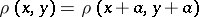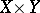# Closed-graph theorem

(diff) ← Older revision | Latest revision (diff) | Newer revision → (diff)
Letandbe complete metric linear spaces with translation-invariant metrics, i.e.,(similarly for), and letbe a linear operator fromto. If the graphof this operator is a closed subset of the Cartesian product, thenis continuous. The closed-graph theorem has various generalizations; for example: a linear mapping with closed graph from a separable barrelled space into a perfectly-complete space is continuous. Closely related theorems are the open-mapping theorem and Banach's homeomorphism theorem.